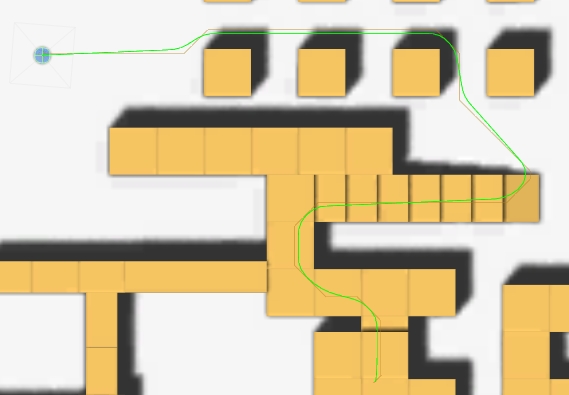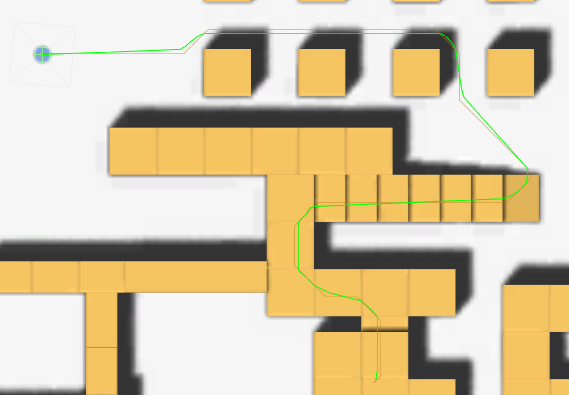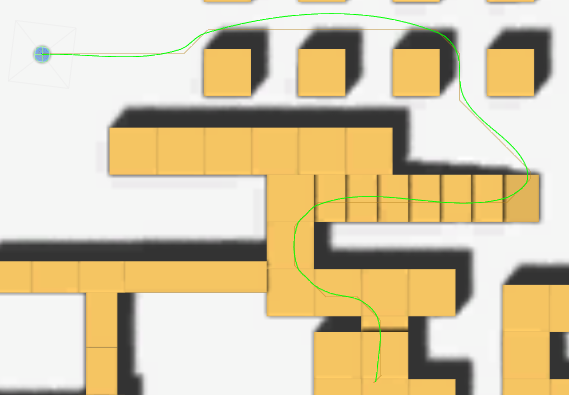A* Pathfinding Project  3.8 The A* Pathfinding Project for Unity 3D
SimpleSmoothModifier Class Reference

Modifier which smooths the path. More...

## Detailed Description

Modifier which smooths the path.

This modifier can smooth a path by either moving the points closer together (Simple) or using Bezier curves (Bezier).

Attach this component to the same GameObject as a Seeker component.
This component will hook in to the Seeker's path post-processing system and will post process any paths it searches for. Take a look at the Modifier Priorities settings on the Seeker component to determine where in the process this modifier should process the path.

Several smoothing types are available, here follows a list of them and a short description of what they do, and how they work. But the best way is really to experiment with them yourself.

• Simple Smooths the path by drawing all points close to each other. This results in paths that might cut corners if you are not careful. It will also subdivide the path to create more more points to smooth as otherwise it would still be quite rough.• Bezier Smooths the path using Bezier curves. This results a smooth path which will always pass through all points in the path, but make sure it doesn't turn too quickly.• OffsetSimple An alternative to Simple smooth which will offset the path outwards in each step to minimize the corner-cutting. But be careful, if too high values are used, it will turn into loops and look really ugly.
• Curved Non UniformNote
Modifies vectorPath array
Todo:
Make the smooth modifier take the world geometry into account when smoothing

## Public Types

enum  SmoothType { Simple, Bezier, OffsetSimple, CurvedNonuniform }

## Public Member Functions

override void Apply (Path p)
Main Post-Processing function.

List< Vector3 > CurvedNonuniform (List< Vector3 > path)

List< Vector3 > SmoothBezier (List< Vector3 > path)

List< Vector3 > SmoothOffsetSimple (List< Vector3 > path)

List< Vector3 > SmoothSimple (List< Vector3 > path)Public Member Functions inherited from MonoModifier
void Awake ()
Alerts the Seeker that this modifier exists.

void OnDestroy ()

void OnDisable ()

void OnEnable ()

virtual void PreProcess (Path p)Public Member Functions inherited from IPathModifier
void Apply (Path p)

void PreProcess (Path p)

## Static Public Member Functions

static Vector3 GetPointOnCubic (Vector3 a, Vector3 b, Vector3 tan1, Vector3 tan2, float t)

## Public Attributes

float bezierTangentLength = 0.4F
Length factor of the bezier curves' tangents'.

float factor = 0.1F
Roundness factor used for CurvedNonuniform.

int iterations = 2
Number of times to apply smoothing.

float maxSegmentLength = 2F
The length of the segments in the smoothed path when using uniformLength.

float offset = 0.2F
Offset to apply in each smoothing iteration when using Offset Simple.

SmoothType smoothType = SmoothType.Simple
Type of smoothing to use.

float strength = 0.5F
Determines how much smoothing to apply in each smooth iteration.

int subdivisions = 2
Number of times to subdivide when not using a uniform length.

bool uniformLength = true
Toggle to divide all lines in equal length segments.Public Attributes inherited from MonoModifier
Seeker seeker

## Properties

override int Order` [get]`Properties inherited from MonoModifier
abstract int Order` [get]`
Modifiers will be executed from lower order to higher order.Properties inherited from IPathModifier
int Order` [get]`

## Member Enumeration Documentation

 enum SmoothType
Enumerator:
 Simple Bezier OffsetSimple CurvedNonuniform

## Member Function Documentation

 override void Apply ( Path p )
virtual

Main Post-Processing function.

Implements MonoModifier.

 List CurvedNonuniform ( List< Vector3 > path )
 static Vector3 GetPointOnCubic ( Vector3 a, Vector3 b, Vector3 tan1, Vector3 tan2, float t )
static
 List SmoothBezier ( List< Vector3 > path )
 List SmoothOffsetSimple ( List< Vector3 > path )
 List SmoothSimple ( List< Vector3 > path )

## Member Data Documentation

 float bezierTangentLength = 0.4F

Length factor of the bezier curves' tangents'.

 float factor = 0.1F

Roundness factor used for CurvedNonuniform.

 int iterations = 2

Number of times to apply smoothing.

 float maxSegmentLength = 2F

The length of the segments in the smoothed path when using uniformLength.

A high value yields rough paths and low value yields very smooth paths, but is slower

 float offset = 0.2F

Offset to apply in each smoothing iteration when using Offset Simple.

smoothType
 SmoothType smoothType = SmoothType.Simple

Type of smoothing to use.

 float strength = 0.5F

Determines how much smoothing to apply in each smooth iteration.

0.5 usually produces the nicest looking curves.

 int subdivisions = 2

Number of times to subdivide when not using a uniform length.

 bool uniformLength = true

Toggle to divide all lines in equal length segments.